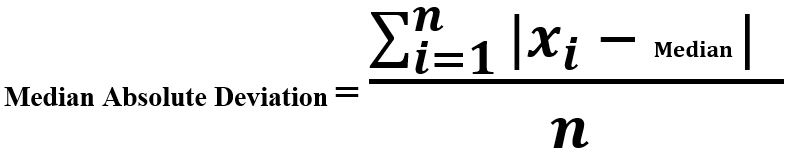# Estimation of Variable | set 2

Prerequisite: Estimation of Variable | set 1

Terms related to Variability Metrics :

```-> Deviation
-> Variance
-> Standard Deviation
-> Mean Absolute Deviation
-> Order Statistics
-> Range
-> Percentile
-> Inter-quartile Range
```
• Median Absolute Deviation : Mean Absolute Devation, Variance and Standard deviation (discussed in previous section) are not robust to extreme values and outliers. We average the sum of deviations from the median.Example :

```Sequence : [2, 4, 6, 8]
Mean     = 5
Deviation around mean = [-3, -1, 1, 3]

Mean Absolute Deviation = (3 + 1 + 1 + 3)/ 4
```

 `# Median Absolute Deviation ` ` `  `import` `numpy as np ` ` `  `def` `mad(data): ` `    ``return` `np.median(np.absolute( ` `            ``data ``-` `np.median(data))) ` `     `  `Sequence ``=` `[``2``, ``4``, ``10``, ``6``, ``8``, ``11``]  ` ` `  `print` `(``"Median Absolute Deviation : "``, mad(Sequence)) ` `    `

Output :

`Median Absolute Deviation :  3.0`
• Order Statistics : This variability measurement approach is based on spread of ranked (sorted) data.
• Range : It is the most basic measurement belonging to Order Statistics. It is the difference between the largest and the smallest value of the dataset. It is good to know the spread of data but it is very sensitive to outliers. We can make it better by dropping the extreme values.
Example :

```Sequence : [2, 30, 50, 46, 37, 91]
Here, 2 and 91 are outliers

Range = 91 - 2 = 89
Range without outliers = 50 - 30 = 20
```
• Percentile: It is the a very good measure to measure the variablity in data, avoiding the outliers. Pth percentile in data is a value such that altleast P% or less values are lesser than it and atleast (100 – P)% values are more than P.
Median is the 50th percentile of the data.
Example :

```Sequence : [2, 30, 50, 46, 37, 91]
Sorted   : [2, 30, 37, 46, 50, 91]

50th percentile = (37 + 46) / 2 = 41.5
```

Code –

 `# Percentile ` ` `  `import` `numpy as np ` ` `  `     `  `Sequence ``=` `[``2``, ``30``, ``50``, ``46``, ``37``, ``91``]  ` ` `  `print` `(``"50th Percentile : "``, np.percentile(Sequence, ``50``)) ` `     `  `print` `(``"60th Percentile : "``, np.percentile(Sequence, ``60``)) `

Output :

```50th Percentile :  41.5
60th Percentile :  46.0```
• Inter-Quartile Range(IQR) : It works for the ranked(sorted data). It has 3 quartiles dividing data – Q1(25th percentile), Q2(50th percentile) and Q3(75th percentile). Inter-quartile Range is the difference between Q3 and Q1.

Example :

```Sequence : [2, 30, 50, 46, 37, 91]
Q1 (25th percentile) : 31.75
Q2 (50th percentile) : 41.5
Q3 (75th percentile) : 49

IQR = Q3 - Q1 = 17.25
```

Code – 1

 `# Inter-Quartile Range ` ` `  `import` `numpy as np ` `from` `scipy.stats ``import` `iqr ` `     `  `Sequence ``=` `[``2``, ``30``, ``50``, ``46``, ``37``, ``91``]  ` ` `  `print` `(``"IQR : "``, iqr(Sequence)) `

Output :

```IQR :  17.25
```

Code – 2

 `import` `numpy as np ` ` `  `# Inter-Quartile Range ` `iqr ``=` `np.subtract(``*``np.percentile(Sequence, [``75``, ``25``])) ` ` `  `print` `(``"\nIQR : "``, iqr) `

Output :

```IQR :  17.25
```

• My Personal Notes arrow_drop_upIf you like GeeksforGeeks and would like to contribute, you can also write an article using contribute.geeksforgeeks.org or mail your article to contribute@geeksforgeeks.org. See your article appearing on the GeeksforGeeks main page and help other Geeks.

Please Improve this article if you find anything incorrect by clicking on the "Improve Article" button below.

Article Tags :
Practice Tags :

Be the First to upvote.

Please write to us at contribute@geeksforgeeks.org to report any issue with the above content.